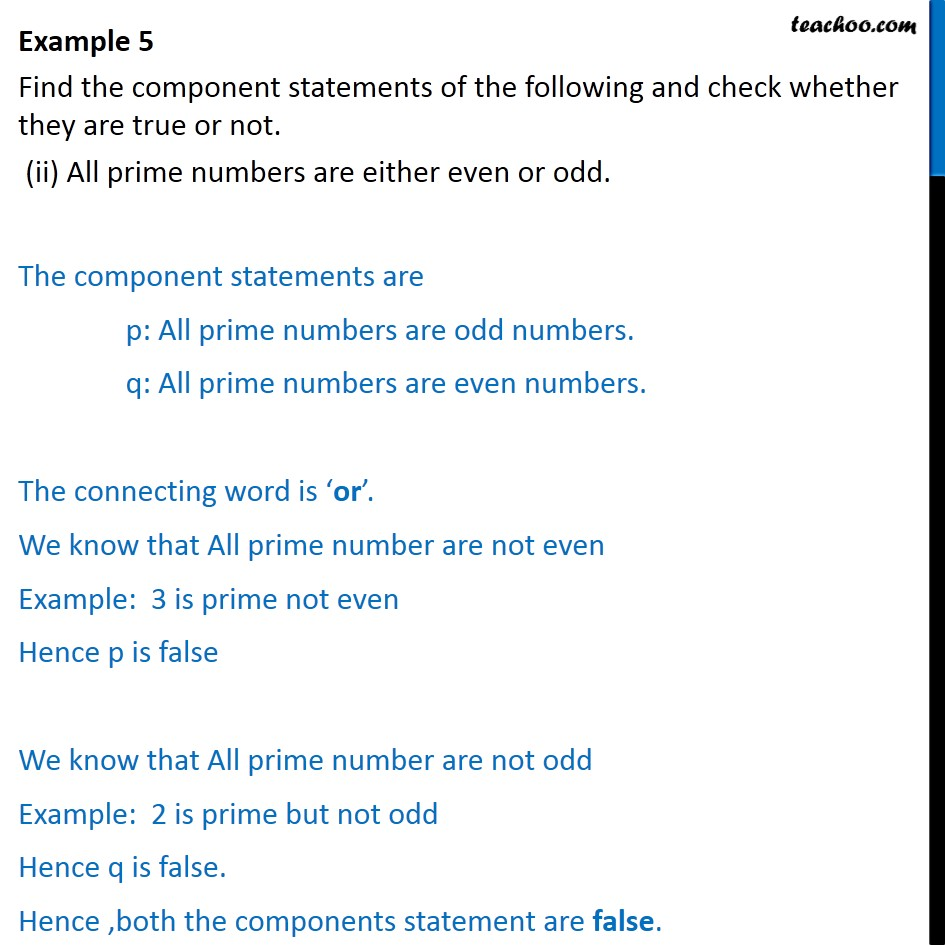Examples

Chapter 14 Class 11 Mathematical Reasoning
Serial order wiseIntroducing your new favourite teacher - Teachoo Black, at only ₹83 per month

### Transcript

Example 5 Find the component statements of the following and check whether they are true or not. (ii) All prime numbers are either even or odd. The component statements are p: All prime numbers are odd numbers. q: All prime numbers are even numbers. The connecting word is or . We know that All prime number are not even Example: 3 is prime not even Hence p is false We know that All prime number are not odd